# Bridging comparative statics and dynamics in Samuelson’s 1941 IS-LM model

This post is based on parts of chapter 7 of our book that have already been published here. All comments are welcomed.

Samuelson argued in 1941 that “a belief in the validity of the law of markets was a necessary badge of membership in the classical school of political economy, and comprehension of its logical derivation formed the pons assinorum for the budding theorist” (Econometric Society, 1941: 177). The expression “pons assinorum” or “bridge of asses,” dating back to Euclid’s Elements, was a way for Samuelson to underline the widely accepted character of the law of markets but also to mock those who failed to understand it. The same year, his own paper on stability analysis of aggregate systems built its own pons assinorum, when he provided the long awaited link between comparative statics and dynamic analysis in a Keynesian system (see this previous post on Meade).

Soon after investigating the effects of public expenditures on the basis of the multiplier-accelerator model (see this previous post), Samuelson shifted gears and revisited the issue on the basis of a simple IS-LM model presenting the interaction between the money and goods markets, based on the works of Hicks (1937), Meade (1937) and Lange (1938). While Meade had already tried to tackle the problem of stability, he had concluded that such a problem could be addressed on the basis of a static model from which stability conditions could be derived through the examination of different elasticities. This was criticized by the econometricians, and Samuelson offered in 1941 the first way to derive “meaningful theorems” from explicitly stated dynamic stability conditions.

There are important differences between the multiplier-accelerator (M-A) model and the IS-LM model. First, while monetary factors play no role in the M-A model they are central in the IS-LM model. This is because in the IS-LM model, investment depends among other things on the rate of interest determined conjointly by the LM and IS blocks. Second, while equilibrium between aggregate demand and aggregate supply is assumed to be reached permanently in the M-A model, this happens to be the case only when the economy has reached its stationary state in the IS-LM model.

With the aim to study the effect of various policies, Samuelson starts from a system of three equations

(1)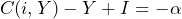(2)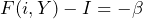(3)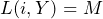wherestands for the interest rate,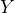, for real income and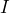for investment output;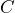for the consumption function,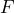for the-marginal-efficiency-of capital schedule,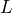for the schedule of liquidity preference,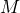for the constant existing amount of money, and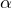and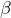for two parameters responsible for upward or downward shifts in the propensity-to-consume and the marginal-efficiency schedules. He thus obtained a static system of three equations and three unknowns in terms of three parameters and three unspecified functions.

Equation (1) defines the equality between aggregate demand,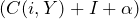, and aggregate supply. Equation (2) defines investmentas as the sum of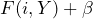. The combination of equations (1) and (2) leads to the IS curve, representing all the combinations of income and interest rate for which production is equal to demand on the goods market (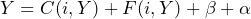). The third equation is the equation of the LM curve, that is, all the combination (,) for which the money market is at equilibrium. Provided that the system has a solution, one can express the equilibrium values of income and interest rate as functions of the parameters,and.

(4)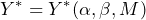(5)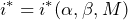Samuelson then asked what would be the reaction of the equilibrium values of income and the interest rate to a shift in the parameters,and. If we consider for instance the effects of a shift in government expenditures, and by totally differentiating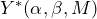and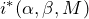with respect tobut assuming thatandremain unchanged, we have:

(6)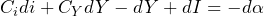(7)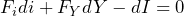(8)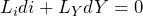with the solutions for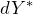and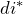:

(9)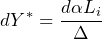(10)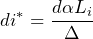where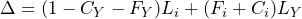On the basis of a priori behavioral assumptions on investment and consumption, it is possible to determine the sign of the parameters of the economy (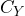,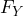, …). This is however not the case for the sign of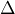which is an ambiguous combination of those different parameters.

Samuelson’s argument is that an analysis of the explicitly dynamic process of convergence to equilibrium in this system can tell us something about the sign of this determinant, through the assumption that the equilibrium will be stable. He introduced two possible adjustment processes, one in continuous time and one in discrete time; we reproduce below the continuous time system where the changes in income depend on the difference “between intended savings-investment and actual savings-investment” (Samuelson, 1941: 116):

(11)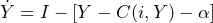(12)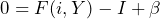(13)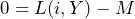This system is very similar to the static one with the difference that a one-dimensional dynamic adjustment process is now added. If we linearise the equations, and replace (12) and (13) in (11), we obtain a non-homogeneous differential equation of the first order inof the form:

(14)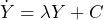where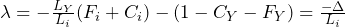andis a constant determined by the parameters of the system. Since the stability of the differential equation depends on the fact that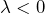, and since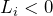(an increase in the interest rate decreases the demand for money), this means that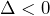for the equilibrium to be stable. This is how, from a consideration of the dynamics of the system, the sign of the ambiguous determinant of the static system is resolved.

Most importantly, it is only at that condition that any static comparative exercises tell us something meaningful about the reaction of the economy once the parameter has changed. If the equilibrium is unstable, the new position of equilibrium of the economy would tell us nothing about the effective movement of income and the interest rate, because the economy will never attain this new position. At the end of the day, there is thus a need to ensure that the equilibrium is stable before making any static comparative exercises. This explains why Samuelson never made any static comparative exercises related to money wages on the basis of the IS-LM model. To do otherwise would have meant to assume full employment equilibrium stability for flexible wages, an assumption that Samuelson was not ready to make.

The following application reproduces the argument exposed above in two panels: one of the familiar IS-LM diagram and the other of the trajectory of income, the interest rate and investment. The trajectory of the economy in the phase plane determined by income and the interest rate also appears in the first panel; when unstable parameters are chosen, it clearly appears that the trajectory does not converge to the new equilibrium.

References:

Econometric Society. 1941. “Report of the Chicago Meeting, December 27-28, 1940, and of the New Orleans Meeting, December 27-30, 1940.” Econometrica 9(2):165–84.

Hicks, John R. 1937. “Mr. Keynes and the” Classics”; a Suggested Interpretation.” Econometrica: Journal of the Econometric Society 5(2):147–59.

Lange, Oskar. 1938. “The Rate of Interest and the Optimum Propensity to Consume.” Economica 5(17):12–32.

Meade, J. E. 1937. “A Simplified Model of Mr. Keynes’ System.” The Review of Economic Studies 4(2):98.

Samuelson, Paul A. 1941. “The Stability of Equilibrium: Comparative Statics and Dynamics.” Econometrica 9(2): 97–120.